TAG: Algebra
April 25, 2011–

Wolfram|Alpha is written in Mathematica, which as its name suggests is a fantastic system for doing mathematics. Strong algorithms for algebraic simplification have always been a central feature of computer algebra systems, so it should come as no surprise to know that Mathematica excels at simplifying algebraic expressions. The main two commands for simplifying an expression in Mathematica are Simplify and FullSimplify. There are also many specific commands for expressing an algebraic expression in some form. For example, if you want to expand a product of linear polynomials, Expand is the appropriate function.

The good news is that everyone has access to the power of Mathematica‘s simplification and algebraic manipulation commands in Wolfram|Alpha. We will now outline some of these features in Wolfram|Alpha, starting with the expression: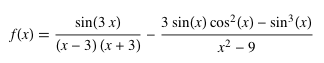and we will use Wolfram|Alpha to break it down to something significantly much simpler.
More »

January 12, 2011–

Today we’re releasing the first three of a planned series of “course assistant” apps, built using Wolfram|Alpha technology.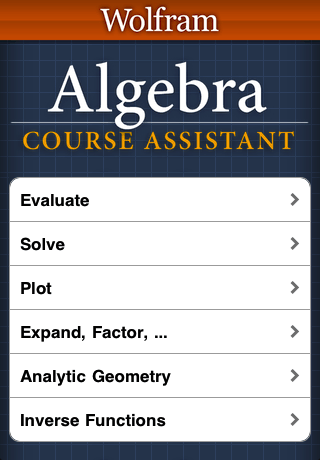The long-term goal is to have an assistant app for every major course, from elementary school to graduate school. And the good news is that Wolfram|Alpha has the breadth and depth of capabilities to make this possible—and not only in traditionally “computational” kinds of courses.

The concept of these apps is to make it as quick and easy as possible to access the particular capabilities of Wolfram|Alpha relevant for specific courses. Each app is organized according to the major curriculum units of a course. Then within each section of the app, there are parts that cover each of the particular types of problems relevant to that unit.
More »

May 7, 2010–

Hello, fellow readers of the Wolfram|Alpha Blog—my name’s Justin. In just a few short weeks, I’ll be graduating from the University of Illinois at Urbana-Champaign. Over the years I’ve found my own way of getting things done in regards to homework and studying routines. But this semester I realized there were tools available that would make studying and completing assignments easier and help me understand better. One tool that has become increasingly valuable in my routine and those of other students on my campus is Wolfram|Alpha. Recently, I was invited to share how Wolfram|Alpha is being used by students like myself.

Being a marketing major, I had to take some finance and accounting courses, but I was a bit rusty with the required formulas and the overall understanding of the cash flow concepts, such as future cash flows and the net present values of a future investment. A friend recommended I check out Wolfram|Alpha’s finance tools, and they’ve became indispensable in my group’s casework for the semester. Each proposed future investment we were met with, we would go directly to Wolfram|Alpha to compute the cash flows. We even went as far to show screenshots, such as the one below, of inputs and outputs in our final case presentation last week.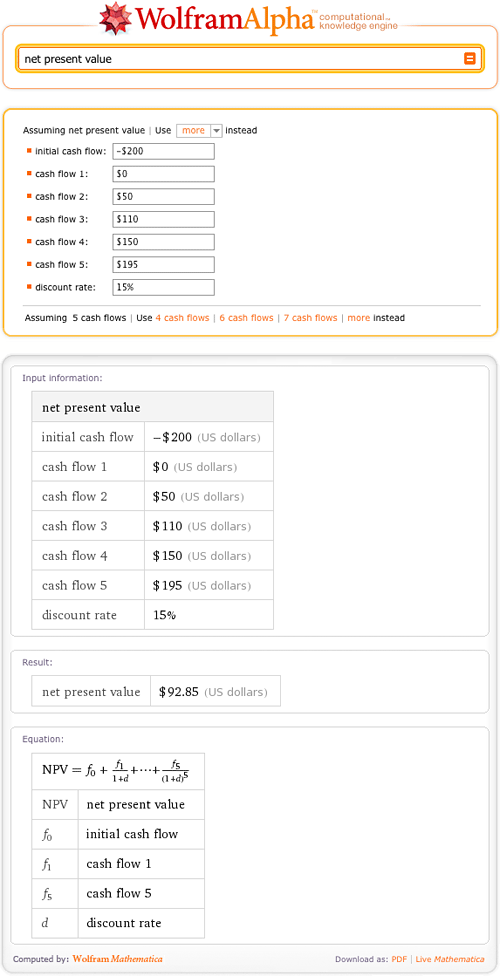I’ve met other students on my campus who have found Wolfram|Alpha to be helpful in their courses. A few months ago while studying in the library, I walked by a table of freshman students all using Wolfram|Alpha on their laptops. I decided to stop and chat with them because I knew one of the girls. They explained how they were using Wolfram|Alpha to model functions and check portions of their math homework. All three girls are enrolled in Calculus III, and not exactly overjoyed about the fact of future— and most likely harder—math classes. More »

March 19, 2010–

Steven Strogatz, a professor of applied mathematics at Cornell University, is currently blogging for The New York Times about issues “from the basics of math to the baffling”. It’s been a fascinating series, starting with preschool math and progressing through subtraction, division, complex numbers, and more. As Wolfram|Alpha is such a powerful tool for working with mathematical concepts, we thought it’d be fun to show how to use it to explore some of the topics in Strogatz’s blog.

First up is Strogatz’s post on “Finding Your Roots”. For a brief introduction to Wolfram|Alpha’s ability to find roots, try “root of 4x+2”.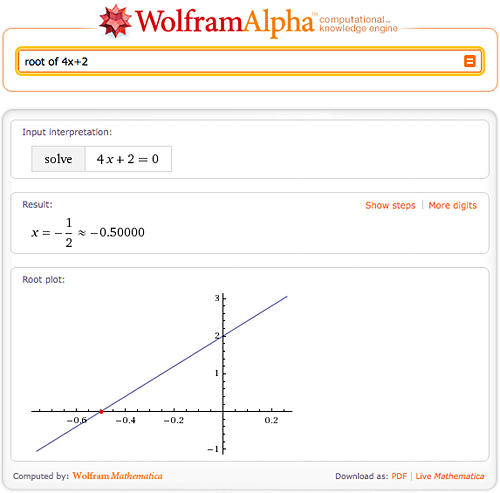Here we found the one and only root of 4x+2, but what if there is more than one root? Not a problem for Wolfram|Alpha—try “4x^2 + 3x – 4”. More »

January 14, 2010–

Need a tutor for solving equations? Solving equations is just one of hundreds of mathematical tasks that can be done using Wolfram|Alpha. Wolfram|Alpha can solve equations from middle school level all the way through college level and beyond. So next time you are stumped on an equation, consult Wolfram|Alpha for a little help.

Let’s start with the simpler stuff. Wolfram|Alpha can easily solve linear and quadratic equations, and even allows you to view a step-by-step solution of each problem.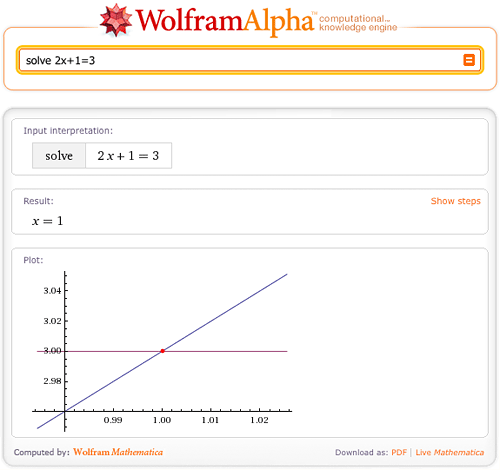What if the roots of the equation are complex? No worries; Wolfram|Alpha has no trouble solving equations over the complex plane.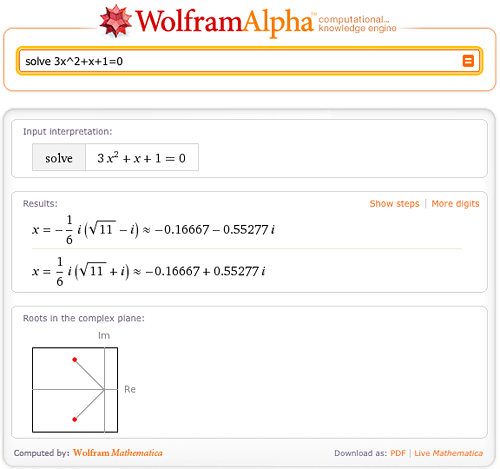Wolfram|Alpha can also solve cubic and quartic equations in terms of radicals.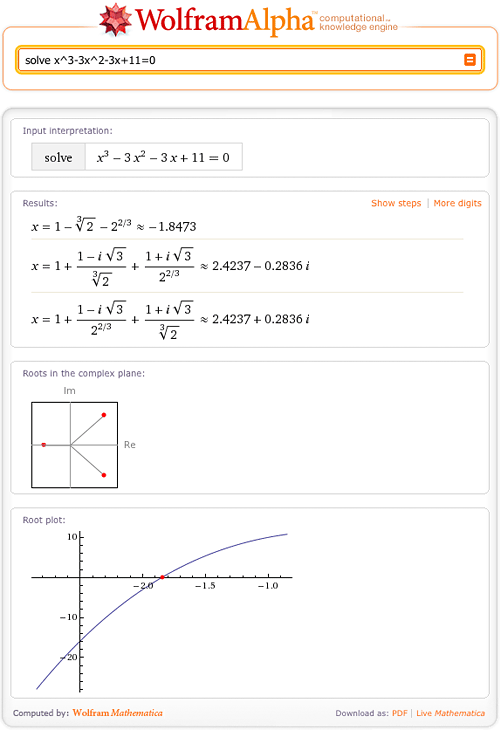Of course, some solutions are too large or cannot be represented in terms of radicals; Wolfram|Alpha will then return numerical solutions with a “More digits” button. More »

December 21, 2009–

When we launched Wolfram|Alpha in May 2009, it already contained trillions of pieces of information—the result of nearly five years of sustained data-gathering, on top of more than two decades of formula and algorithm development in Mathematica. Since then, we’ve successfully released a new build of Wolfram|Alpha’s codebase each week, incorporating not only hundreds of minor behind-the-scenes enhancements and bug fixes, but also a steady stream of major new features and datasets.

We’ve highlighted some of these new additions in this blog, but many more have entered the system with little fanfare. As we near the end of 2009, we wanted to look back at seven months of new Wolfram|Alpha features and functionality.